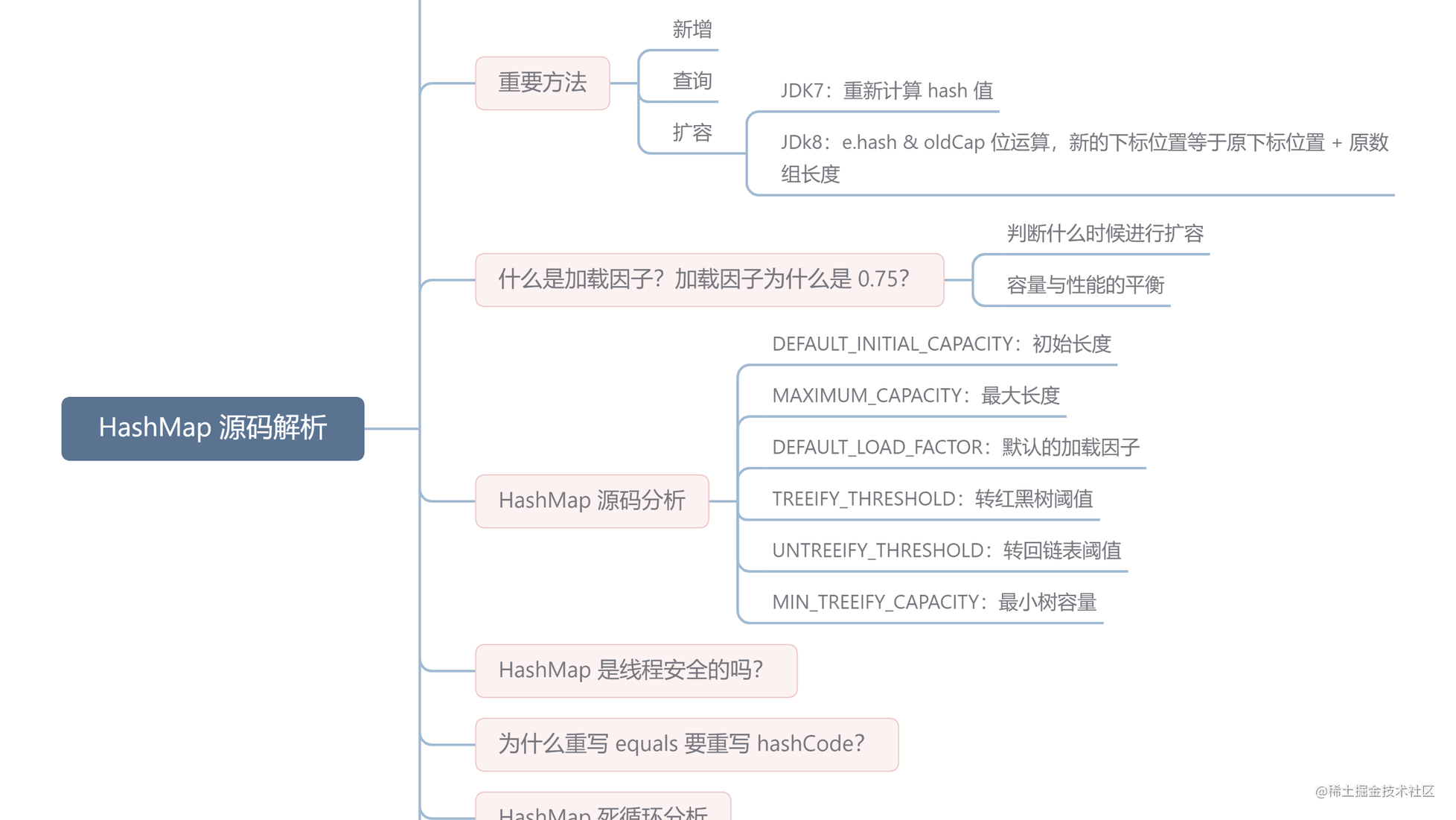# 工作三年，小胖连 HashMap 源码都没读过？真的菜！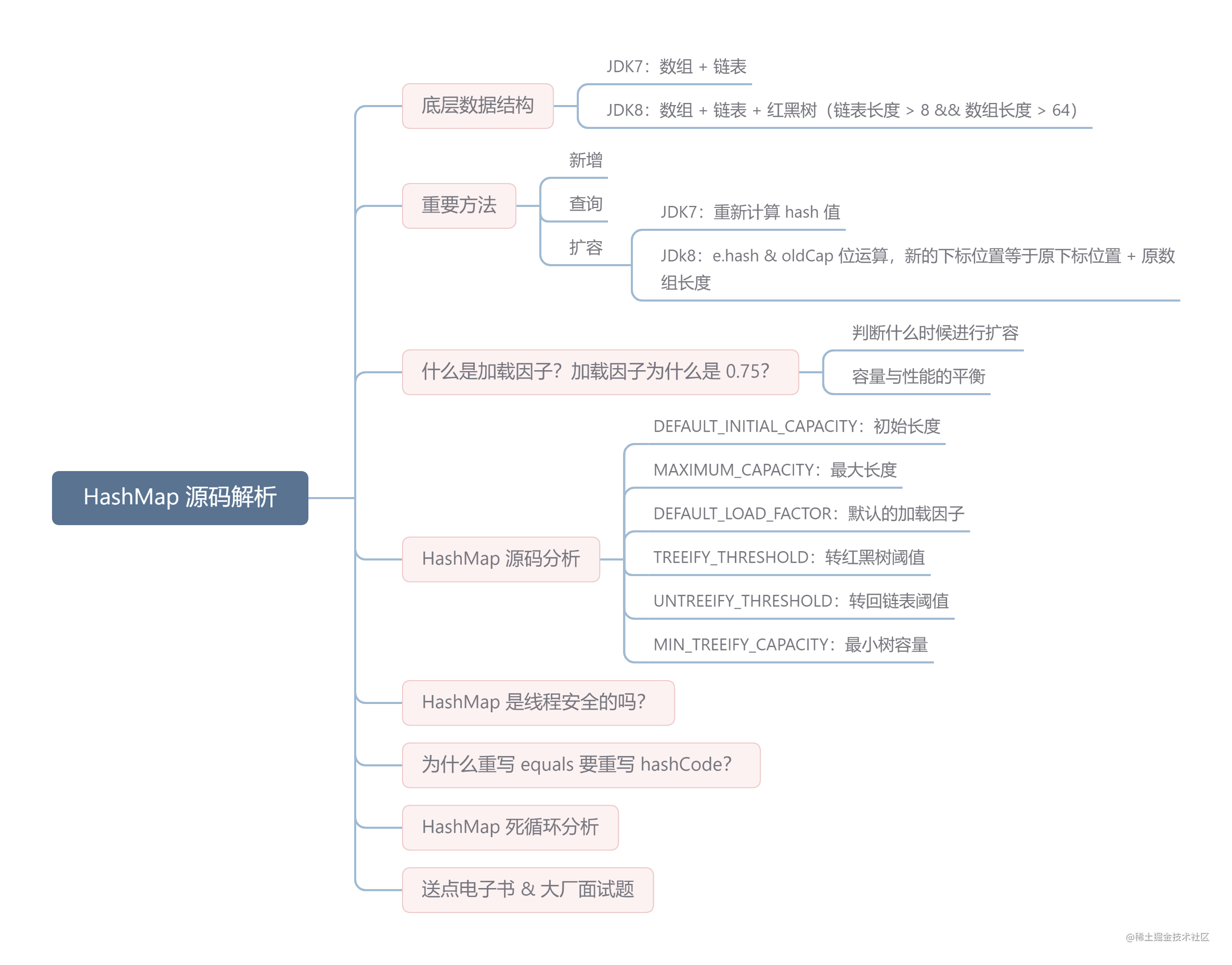# 00 HashMap 的底层数据结构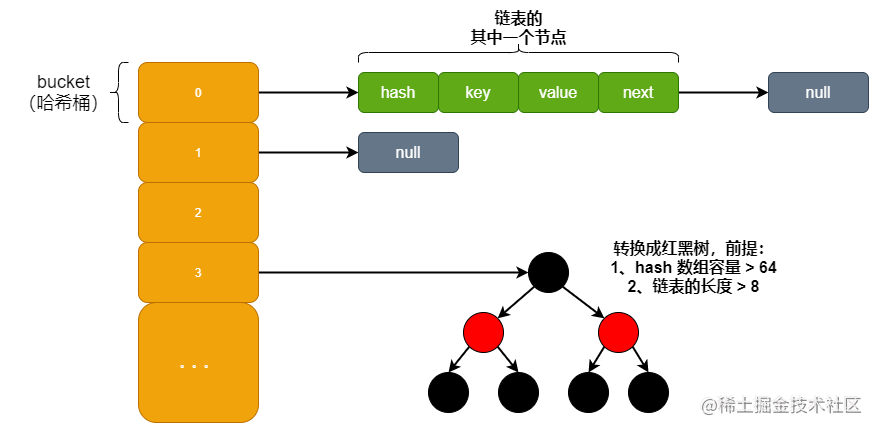HashMap 中数组的每一个元素又称为哈希桶，也就是 key-value 这样的实例。在 Java7 中叫 Entry，Java8 中叫 Node。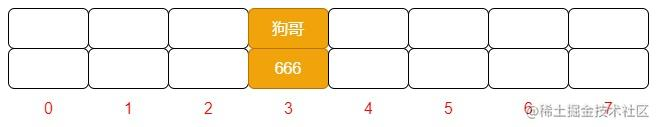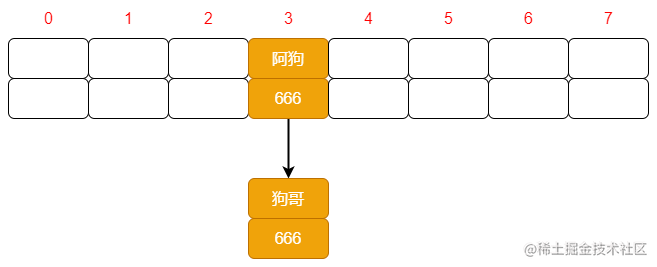``````static class Node < K, V > implements Map.Entry < K, V > {
final int hash;
final K key;
V value;
Node < K,
V > next;

...
}

JDK 1.8 之所以添加红黑树是因为一旦链表过长，会严重影响 HashMap 的性能，而红黑树具有快速增删改查的特点，这样就可以有效的解决链表过长时操作比较慢的问题。

# 01 HashMap 的重要方法

PS：以下源码分析全部基于 JDK1.8 版本。

## 1.0 查询（get 方法）

``````public V get(Object key) {
Node < K, V > e;
// 对 key 进行哈希操作
return (e = getNode(hash(key), key)) == null ? null : e.value;
}

final Node < K, V > getNode(int hash, Object key) {
Node < K, V > [] tab;
Node < K, V > first, e;
int n;
K k;
// 非空判断
if ((tab = table) != null && (n = tab.length) > 0 &&
(first = tab[(n - 1) & hash]) != null) {
// 判断第一个元素是否是要查询的元素
// always check first node
if (first.hash == hash &&
((k = first.key) == key || (key != null && key.equals(k))))
return first;
// 下一个节点非空判断
if ((e = first.next) != null) {
// 如果第一节点是树结构，则使用 getTreeNode 直接获取相应的数据
if (first instanceof TreeNode)
return ((TreeNode < K, V > ) first).getTreeNode(hash, key);
do { // 非树结构，循环节点判断
// hash 相等并且 key 相同，则返回此节点
if (e.hash == hash &&
((k = e.key) == key || (key != null && key.equals(k))))
return e;
} while ((e = e.next) != null);
}
}
return null;
}

## 1.1 新增（put 方法）

``````public V put(K key, V value) {
// 对 key 进行哈希操作
return putVal(hash(key), key, value, false, true);
}

final V putVal(int hash, K key, V value, boolean onlyIfAbsent,
boolean evict) {
Node < K, V > [] tab;
Node < K, V > p;
int n, i;
// 哈希表为空则创建表
if ((tab = table) == null || (n = tab.length) == 0)
n = (tab = resize()).length;
// 根据 key 的哈希值计算出要插入的数组索引 i
if ((p = tab[i = (n - 1) & hash]) == null)
// 如果 table[i] 等于 null，则直接插入
tab[i] = newNode(hash, key, value, null);
else {
Node < K, V > e;
K k;
// 如果 key 已经存在了，直接覆盖 value
if (p.hash == hash &&
((k = p.key) == key || (key != null && key.equals(k))))
e = p;
// 如果 key 不存在，判断是否为红黑树
else if (p instanceof TreeNode)
// 红黑树直接插入键值对
e = ((TreeNode < K, V > ) p).putTreeVal(this, tab, hash, key, value);
else {
// 为链表结构，循环准备插入
for (int binCount = 0;; ++binCount) {
// 下一个元素为空时
if ((e = p.next) == null) {
p.next = newNode(hash, key, value, null);
// 转换为红黑树进行处理
if (binCount >= TREEIFY_THRESHOLD - 1) // -1 for 1st
treeifyBin(tab, hash);
break;
}
//  key 已经存在直接覆盖 value
if (e.hash == hash &&
((k = e.key) == key || (key != null && key.equals(k))))
break;
p = e;
}
}
// existing mapping for key
if (e != null) {
V oldValue = e.value;
if (!onlyIfAbsent || oldValue == null)
e.value = value;
afterNodeAccess(e);
return oldValue;
}
}
++modCount;
// 超过最大容量，扩容
if (++size > threshold)
resize();
afterNodeInsertion(evict);
return null;
}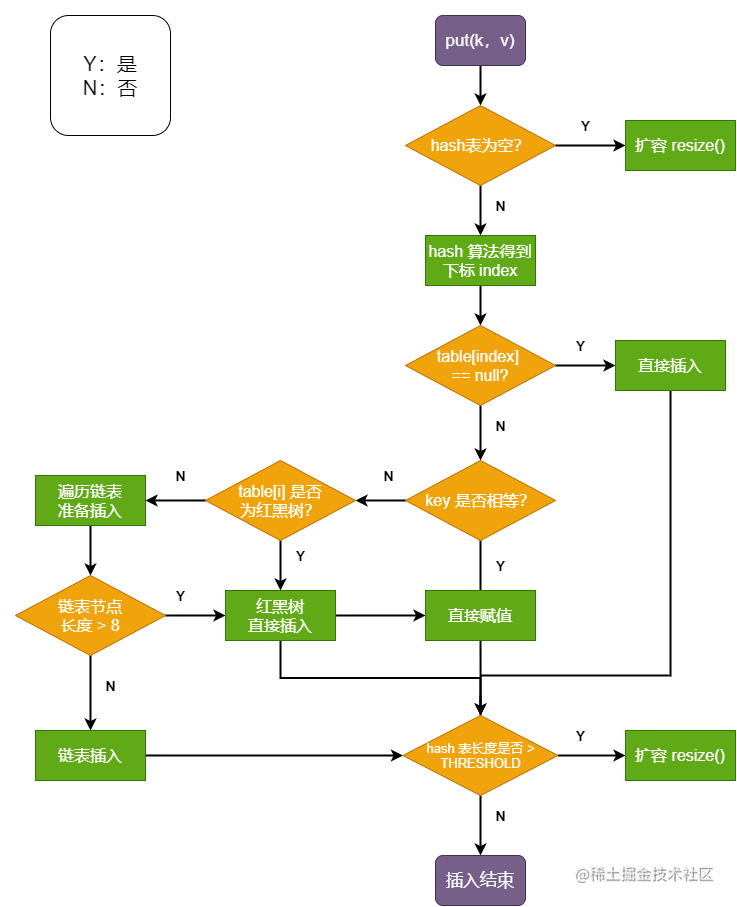## 1.2 扩容（resize 方法）

``````final Node < K, V > [] resize() {
// 扩容前的数组
Node < K, V > [] oldTab = table;
// 扩容前的数组的大小和阈值
int oldCap = (oldTab == null) ? 0 : oldTab.length;
int oldThr = threshold;
// 预定义新数组的大小和阈值
int newCap, newThr = 0;
if (oldCap > 0) {
// 超过最大值就不再扩容了
if (oldCap >= MAXIMUM_CAPACITY) {
threshold = Integer.MAX_VALUE;
return oldTab;
}
// 扩大容量为当前容量的两倍，但不能超过 MAXIMUM_CAPACITY
else if ((newCap = oldCap << 1) < MAXIMUM_CAPACITY &&
oldCap >= DEFAULT_INITIAL_CAPACITY)
newThr = oldThr << 1; // double threshold
}
// 当前数组没有数据，使用初始化的值
else if (oldThr > 0) // initial capacity was placed in threshold
newCap = oldThr;
else { // zero initial threshold signifies using defaults
// 如果初始化的值为 0，则使用默认的初始化容量
newCap = DEFAULT_INITIAL_CAPACITY;
}
// 如果新的容量等于 0
if (newThr == 0) {
float ft = (float) newCap * loadFactor;
newThr = (newCap < MAXIMUM_CAPACITY && ft < (float) MAXIMUM_CAPACITY ?
(int) ft : Integer.MAX_VALUE);
}
threshold = newThr;
@SuppressWarnings({
"rawtypes",
"unchecked"
})
Node < K, V > [] newTab = (Node < K, V > []) new Node[newCap];
// 开始扩容，将新的容量赋值给 table
table = newTab;
// 原数据不为空，将原数据复制到新 table 中
if (oldTab != null) {
// 根据容量循环数组，复制非空元素到新 table
for (int j = 0; j < oldCap; ++j) {
Node < K, V > e;
if ((e = oldTab[j]) != null) {
oldTab[j] = null;
// 如果链表只有一个，则进行直接赋值
if (e.next == null)
newTab[e.hash & (newCap - 1)] = e;
else if (e instanceof TreeNode)
// 红黑树相关的操作
((TreeNode < K, V > ) e).split(this, newTab, j, oldCap);
else { // preserve order
// 链表复制，JDK 1.8 扩容优化部分
// 如果节点不为空，且为单链表，则将原数组中单链表元素进行拆分
Node < K, V > loHead = null, loTail = null;//保存在原有索引的链表
Node < K, V > hiHead = null, hiTail = null;//保存在新索引的链表
Node < K, V > next;
do {
next = e.next;
// 哈希值和原数组长度进行 & 操作，为 0 则在原数组的索引位置
if ((e.hash & oldCap) == 0) {
if (loTail == null)
else
loTail.next = e;
loTail = e;
}
// 原索引 + oldCap
else {
if (hiTail == null)
else
hiTail.next = e;
hiTail = e;
}
} while ((e = next) != null);
// 将原索引放到哈希桶中
if (loTail != null) {
loTail.next = null;
}
// 将原索引 + oldCap 放到哈希桶中
if (hiTail != null) {
hiTail.next = null;
}
}
}
}
}
return newTab;
}

• 扩容：创建一个新的 Entry 空数组，长度是原数组的 2 倍。
• 位运算：原来的元素哈希值和原数组长度进行 & 运算。

JDK 1.8 在扩容时并没有像 JDK 1.7 那样，重新计算每个元素的哈希值，而是通过高位运算（e.hash & oldCap）来确定元素是否需要移动，假设 key1 的信息如下：

• key1.hash = 10；二进制： 0000 1010
• oldCap = 16；二进制： 0001 0000

• key2.hash = 17； 二进制：0001 0001
• oldCap = 16； 二进制：0001 0000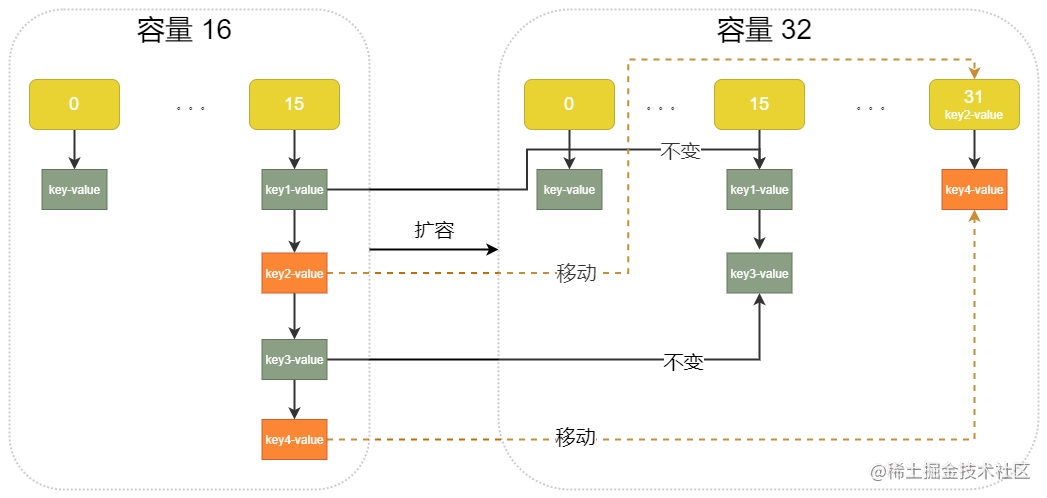# 02 HashMap有哪些属性？

``````// HashMap 初始化长度
static final int DEFAULT_INITIAL_CAPACITY = 1 << 4; // aka 16

// HashMap 最大长度
static final int MAXIMUM_CAPACITY = 1 << 30; // 1073741824

// 默认的加载因子 (扩容因子)
static final float DEFAULT_LOAD_FACTOR = 0.75f;

// 当链表长度大于此值且数组长度大于 64 时，会从链表转成红黑树
static final int TREEIFY_THRESHOLD = 8;

// 转换链表的临界值，当元素小于此值时，会将红黑树结构转换成链表结构
static final int UNTREEIFY_THRESHOLD = 6;

// 最小树容量
static final int MIN_TREEIFY_CAPACITY = 64;

# 05 什么是加载因子？加载因子为什么是 0.75 ？

• 上面说到，为了提升扩容效率，HashMap 的容量（capacity）有一个固定的要求，那就是一定是 2 的幂。所以，如果负载因子是 3/4 的话，那么和 capacity 的乘积结果就可以是一个整数

• 当加载因子设置较大时，扩容门槛提高，扩容发生频率低，占用的空间小，但此时发生 Hash 冲突的几率就会提升，因此需要更复杂的数据结构来存储元素，这样对元素的操作时间就会增加，运行效率也会降低；

• 当加载因子设置较小时，扩容门槛降低，会占用更多的空间，此时元素的存储就比较稀疏，发生哈希冲突的可能性就比较小，因此操作性能会比较高。

# 07 为什么重写 equals 方法的时，需要重写 hashCode 方法呢？

Java 中，所有的对象都是继承于 Object 类。Ojbect 类中有两个方法 equals、hashCode，这两个方法都是用来比较两个对象是否相等的。

``````public boolean equals(Object obj) {
return (this == obj);
}

• 对于值对象，== 比较的是两个对象的值
• 对于引用对象，== 比较的是两个对象的地址

# 08 HashMap 死循环分析

``````void transfer(Entry[] newTable, boolean rehash) {
int newCapacity = newTable.length;
for (Entry < K, V > e: table) {
while (null != e) {
Entry < K, V > next = e.next; // 此处加断点
if (rehash) {
e.hash = null == e.key ? 0 : hash(e.key);
}
int i = indexFor(e.hash, newCapacity);
e.next = newTable[i];
newTable[i] = e;
e = next;
}
}
}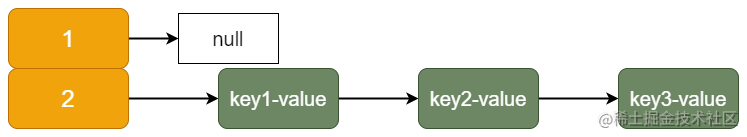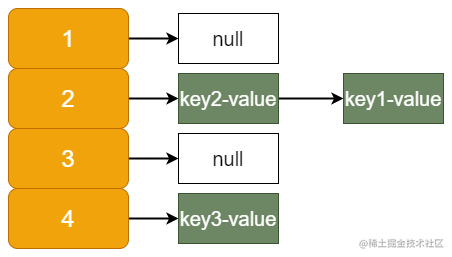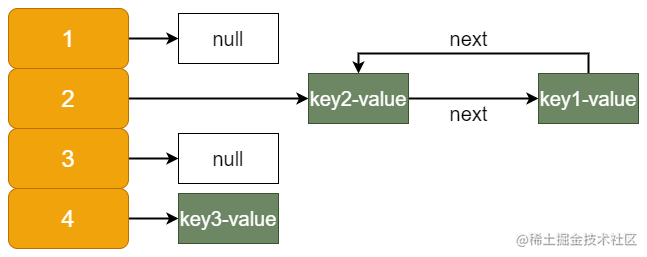# 09 总结

HashMap 是 Java 基础中的重点。可以说无论是在工作中还是面试中都很常用，小伙伴们必须做到熟练运用、信手拈来才算是过关的。本篇文章基本说到了 HashMap 的所有重点，红黑树没有展开说，主要是因为篇幅原因，后面会单独聊。另外，如果发现本文有啥错误，欢迎友善指正。

# 11 送点面试题 & 电子书